# Si0.5Ge0.5¶

This document is part of the QuantumWise Semiconductor Whitepapers. Here we investigate computational methods for ATK-DFT calculations for a simple Si0.5Ge0.5 alloy in the diamond crystal structure, i.e., with an atom of each element in the basis (in this document also denoted SiGe for brevity). We apply the SG15 pseudopotentials and a number of exchange-correlation functionals; PBE, PBEsol, TB09-MGGA, and PBE with the Pseudopotential Projector-Shift method. A limited number of HSE calculatios have been done for comparison. See the section Introduction for more information.

The following quantities have been calculated for each computational method, both at the experimental Si0.5Ge0.5 lattice constant and at the theoretically predicted ones:

• Elastic constants: Bulk modulus, Poisson ratio, and Young’s modulus.
• Band structure along the X–Γ–L Brillouin zone path.
• Direct and indirect band gaps.
• Effective masses in the conduction band minimum.
• Static dielectric constant.

Convergence studies indicate that a 8x8x8 k-point grid and a 100 Hartree density mesh cutoff yields well-converged values for the Si0.5Ge0.5 lattice constant, total energy and indirect band gap. These settings were used for computing the ground state electronic structure used in all the ATK-DFT analyses listed above.

The projector shifts used for the pps-PBE method are 11.23 eV for Si s-orbitals and -1.09 eV for Si p-orbitals, while shifts of 15.0 eV are used for Ge s-orbitals, 0.2 eV for Ge p-orbitals, and $$-$$2.0 eV for Ge d-orbitals. Note that these PPS parameters should only be used with SG15 pseudopotentials.

This document is organized as follows. The section Convergence briefly presents the convergence studies mentioned above, and the section Timing compares CPU timings and scalability of different SG15 basis sets for Si0.5Ge0.5. Next, the section Results contains figures illustrating the performance of the different computational methods in predicting the materials properties listed above. Finally, the Appendix gives a template QuantumATK Python script for setting up ATK-DFT calculations for Si0.5Ge0.5 similar to the ones presented here.

## Summary¶

The following list summarizes the main conclusions from this study.

• The PBEsol, and to a lesser degree pps-PBE, methods both yield very accurate Si0.5Ge0.5 lattice constants, and also give the best results for elastic constants.
• All tested methods yield a qualitatively correct Si0.5Ge0.5 band structure, but with significant differences between the different methods. The method which is closest to the HSE reference is pps-PBE.
• The pps-PBE method accurately reproduces the fundamental (indirect) band gap. There is a large spread in the results for the direct gaps, so care is needed to describe these accurately.
• Electron effective masses in the $$\Delta$$-point CBM are quite well captured by all tested methods.
• Even though PBE and PBEsol do not describe the band gaps as accurately as pps-PBE, they are actually significantly better for the static dielectric constant. It is underestimated by the other methods.

## Convergence¶

The type of Monkhorst–Pack k-point grid and the density mesh cutoff energy are essential ATK-DFT parameters that affect the computational efficiency and precision: A dense k-point grid and high cutoff energy usually give high precision, but may also be computationally intense. It is therefore important to investigate the trade-off between computational precision and cost. Fig. 214 illustrates how the bulk total energy, fundamental band gap, and lattice constant depend on the mesh cutoff and k-point grid.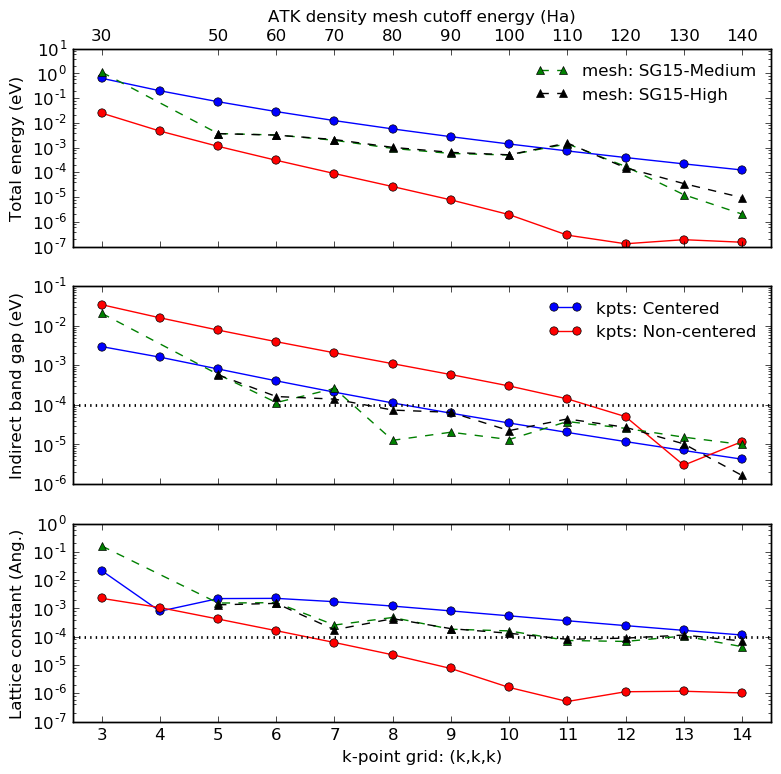Fig. 214 Convergence of the SiGe total energy, fundamental (indirect) band gap, and lattice constant with respect to the ATK-DFT density mesh cutoff (top x-axis in all three panels; green and black dashed lines) and k-point grid (bottom x-axis in all three panels; blue and red lines). The ‘centered’ k-point grids are shifted to the $$\Gamma$$ point (only affects even grids, e.g. 4x4x4), while the ‘non-centered’ k-point grids are shifted by (0.5,0.5,0.5) and therefore do not sample the $$\Gamma$$ point (only affects odd grids, e.g. 3x3x3). The horizontal dotted lines indicate tight convergence criteria.

Note that a standard Monkhorst–Pack k-point grid is Γ-centered for odd numbers of points (e.g. 3x3x3), but does not include the Γ point for even numbers of points (e.g. 4x4x4). The blue lines in Fig. 214 are for k-point grids where even grids are shifted to Γ (such that both 3x3x3 and 4x4x4 grids do include Γ), while the red lines are for calculations where odd grids are shifted away from Γ (such that both 3x3x3 and 4x4x4 grids do not include Γ).

For the SG15 pseudopotential with both Medium and High basis sets, a 100 Hartree mesh cutoff gives band gaps and lattice constants that are converged to within 10-4 eV and roughly 10-4 Å, respectively.

It is also clear that an off-Γ k-point grid (non-centered, red) gives faster convergence of the total energy and lattice constant than the k-point grid that includes the Γ point (centered, blue). We see that the non-centered 8x8x8 Monkhorst–Pack grid yields highly converged lattice constants.

Note

All remaining ATK-DFT calculations in this study therefore use a 100 Hartree mesh cutoff energy and a standard (non-centered) 8x8x8 Monkhorst–Pack k-point grid for both elastic properties and electronic properties.

## Timing¶

The computational cost of a calculation may depend critically on the choice of basis set, particularly for calculations on large systems with many atoms. Fig. 215 shown below illustrates this for the present case of Si0.5Ge0.5 with the Medium, High, and Ultra SG15 basis sets.

The SiGe primitive cell (containing 2 atoms) was systematically repeated in steps of one along all three lattice vectors, resulting in rapidly increasing systems sizes. PBE calculations were then performed for each system, using Γ-point sampling only, to avoid any influence of k-point sampling on the recorded CPU times.

It is quite clear from the figure that the computational loads of the SG15 Medium and High basis sets are not much different for relatively small system sizes, but the High basis set becomes significantly more demanding for larger system sizes. For the 432-atom unit cell, the CPU time difference is roughly a factor of 2. Moreover, the Ultra basis set appears in comparison extremely demanding, and will not be used in the remaining calculations in this study.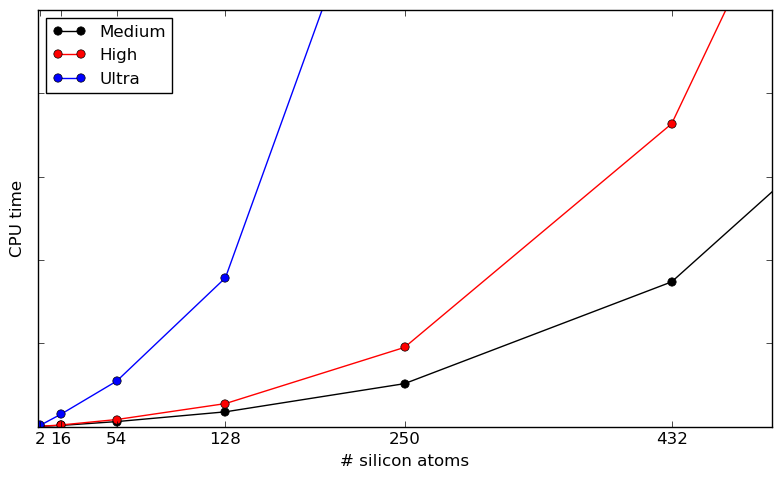Fig. 215 Total CPU time for the first 5 SCF iterations by the ATK-DFT calculator for the Medium (black), High (red), and Ultra (blue) SG15 basis sets, against the number of atoms in the increasingly larger (repeated) SiGe bulk unit cell. Only the Γ point is sampled (1x1x1 k-point grid) and the calculations were executed on a single CPU core. Note that both figure axes are linear.

## Results¶

This section presents results obtained with the PBE, PBEsol, pps-PBE, and TB09-MGGA methods using Medium and High basis sets with the SG15 pseudopotentials for Si0.5Ge0.5.

The cited experimental reference values are in general for a 50/50 mix of Si and Ge, but with no local order in the distribution of Si and Ge on the lattice. There may therefore be a slight difference between the experimentally investigated system and the very small model system used here.

The list below gives direct links to all subsections:

### Lattice constant¶

The PBEsol lattice constant for Si0.5Ge0.5 is very close to the experimental value, 5.538 Å, but the pps-PBE method is also quite accurate. The bare PBE functional (without any pseudopotential projector shifts) is known to overestimate lattice constants in general, and in this case it overestimates by more than 1%. This is in contrast to pure silicon where PBE only overestimates the lattice constant by 0.6%. HSE performs better than PBE, but slightly worse than pps-PBE. However, HSE is expected to perform much better for the details of the electronic structure.

In all cases, except for PBEsol, we see a significantly smaller error for the better basis set (High or Tight).Fig. 216 SiGe lattice constant calculated using the PBEsol (blue), pps-PBE (red), PBE (green), and HSE (black) methods, and two different qualities of basis sets, plotted as deviation from the experimental value from the Ioffe website.

### Elastic constants¶

Both PBESol and pps-PBE hit close to the experimental bulk modulus, while regular PBE underestimates it. The same trend is observed for Young’s modulus, but without a reference value for comparison. The Poisson ratio is approximately the same for all methods.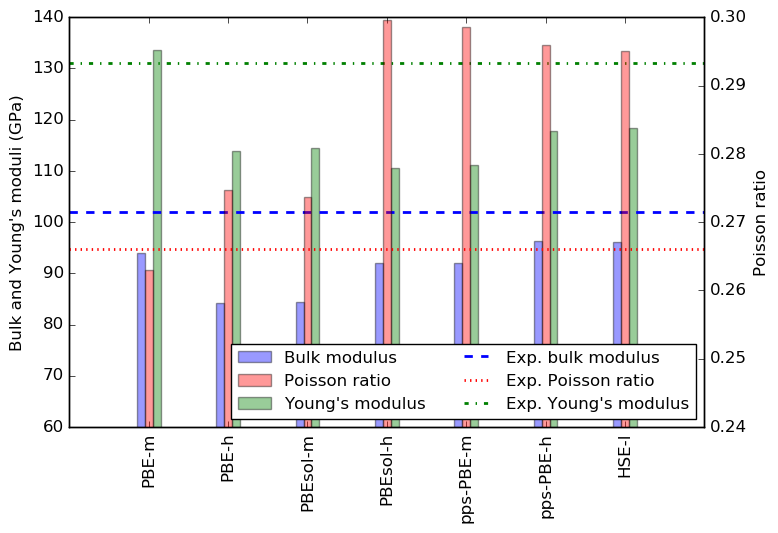Fig. 217 SiGe bulk modulus (blue), Poisson ratio (red), and Young’s modulus (green) calculated using the PBEsol, pps-PBE, and PBE methods, and two different qualities of basis sets. The blue dashed line indicates the experimental bulk modulus, 86.5 GPa, adapted from the Ioffe website.

### Band structure¶

All tested methods correctly predict a valence band maximum (VBM) at the Brillouin zone Γ point, and most also predict a conduction band minimum (CBM) close to the X point, along the Γ–X path. However, the position of the CBM depends slightly on the method applied, and the band energy in the CBM significantly so (see also section Band gaps). In fact, for PBE the CBM may shift to the L point depending on basis set.

There is also a qualitative difference between HSE and pps-PBE at the X point. HSE has a gap of about 0.3 eV between the two lowest conduction bands, while they (almost) touch for pps-PBE.

Changing the basis set from Medium to High gives a shift of about 0.1 eV at the X point, and quickly decreasing towards Γ. In all cases, the shift brings the result closer to the HSE values. For HSE, there is almost no difference between Light and Tight basis sets.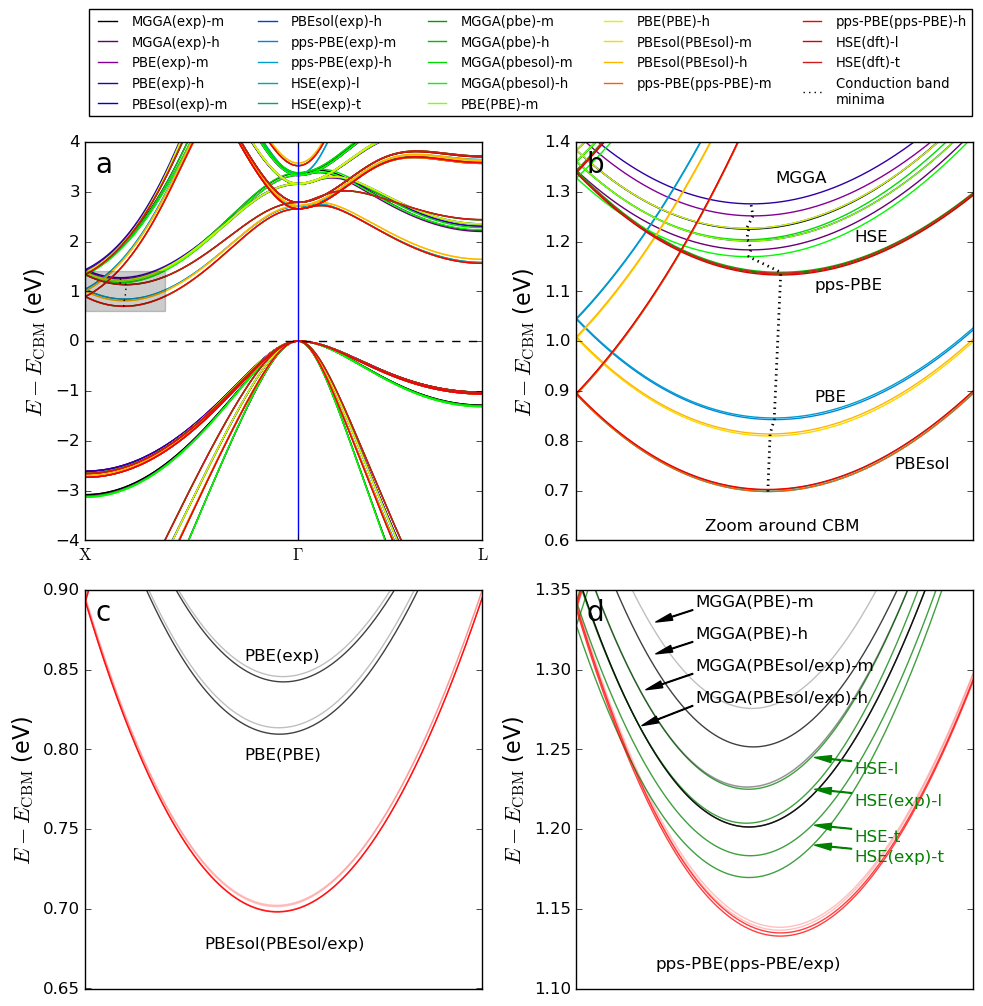Fig. 218 SiGe band structure calculated with the PBE, pps-PBE, PBEsol, TB09-MGGA and HSE exchange-correlation functionals with two different qualities of basis sets. All bandstructures are calculated with the equilibrium structure for that computational model, except for TB09-MGGA, which has been calculated with both the PBE and PBEsol lattice constants.

### Band gaps¶

The SiGe fundamental band gap ($$E_\Delta$$) is especially well reproduced by the pps-PBE method, but also HSE, regular PBE and PBESol give reasonable agreement with experiment. Note, however, that the TB09-MGGA c-parameter was calculated self-consistently from the electronic structure (no fitting), while the pps-PBE projector shifts were essentially fitted to the silicon and germanium band gaps and lattice constants. On the other hand, the TB09-MGGA functional cannot be used for geometry optimization, which the pps-PBE method is well suited for.

There is much less agreement between the methods when it comes to the direct gaps, with TB09-MGGA consistently giving larger values, PBE and PBESol giving smaller values, and pps-PBE and HSE in between.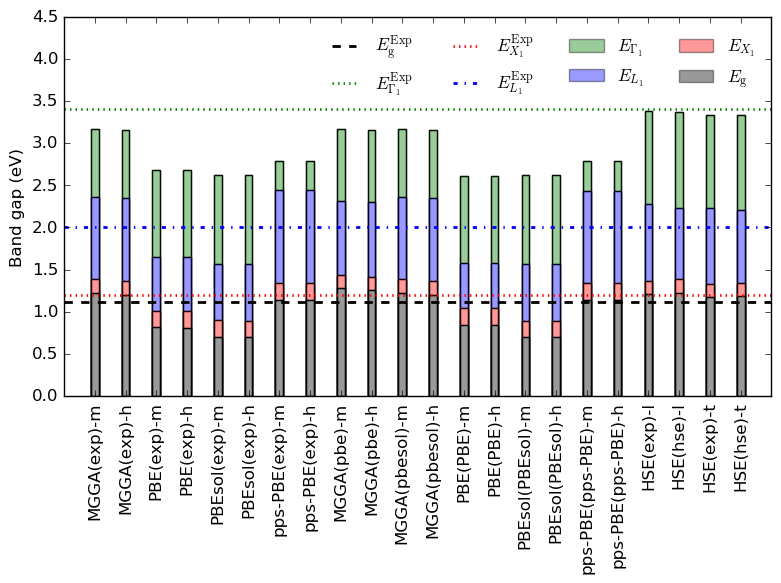Fig. 219 SiGe band gaps, including both the fundamental gap ($$E_\Delta$$, indirect), and the direct gaps to the X, $$\Gamma$$, and L points. The experimental $$E_\Delta$$ of 0.917 eV is from the Ioffe website, and is indicated by a black dashed line.

We also evaluate the effect of using HSE for band gap calculations. The figure below shows GGA and HSE band gaps of SiGe computed at the GGA lattice constants. The HSE indirect (fundamental) gaps are all roughly the same, while the direct gap at the $$\Gamma$$ point does vary with lattice constant. The latter is probably due to strain effects. The PBE and PBEsol gaps are all significantly smaller than the corresponding HSE ones, as expected. On the other hand, the pps-PBE gaps are quite similar to the corresponding HSE gaps.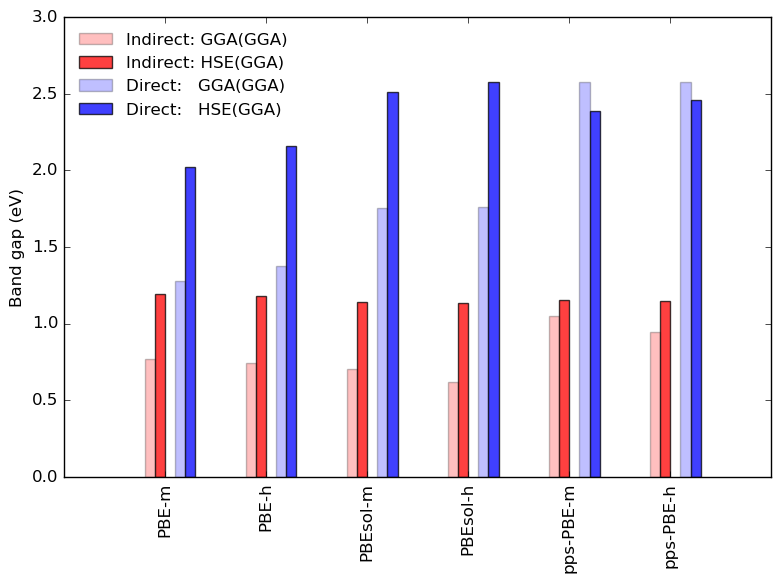Fig. 220 Comparison of GGA and HSE indirect ($$E_\Delta$$) and direct ($$E_{\Gamma_1}$$) band gaps at GGA lattice constants.

### Effective masses¶

The SiGe electron effective masses in the $$\Delta$$-point CBM are fairly close to experimental values for all applied methods.Fig. 221 SiGe transverse (blue; left y-axis) and longitudinal (red; right y-axis) electron effective masses calculated in the $$\Delta$$-point conduction band minimum. Experimental effective masses are from the Ioffe website.

### Dielectric constant¶

The static ($$\omega=0$$) dielectric constant of the SiG lattice is most reliably reproduced by the PBE and PBEsol functionals, with the pps-PBE method a bit off, and TB09-MGGA more than 30% off. The latter is probably related to the fact that TB09-MGGA severely overestimates the direct band gap, while PBE and PBEsol are closer to the experimental direct gap. However, the fact pps-PBE gives a very good direct gap, but is somewhat off for the static dielectric constant, may indicate that the overall bandstructure is not as accurately described as the direct gap itself. We see almost no dependence of the dielectric constant on the basis set quality.Fig. 222 Static dielectric constant of SiGe calculated with PBE, PBEsol, TB09-MGGA and pps-PBE methods, and two qualitites of basis sets. The experimental static dielectric constant of 14.0 is from the Ioffe website.

## Appendix¶

The QuantumATK Python script shown below may be used as a template for ATK-DFT calculations for Si0.5Ge0.5 with the SG15 pseudopotential. The script defines the SiGe bulk configuration and then sets up the ATK-DFT calculator with PBE exchange-correlation. The script blocked named Basis Set shows various options for the SG15 basis set; ordinary PBE and PBE with pseudopotential projector shifts, both with Medium and High basis sets.

The script is available for direct download: si50ge50.py.

  1 2 3 4 5 6 7 8 9 10 11 12 13 14 15 16 17 18 19 20 21 22 23 24 25 26 27 28 29 30 31 32 33 34 35 36 37 38 39 40 41 42 43 44 45 46 47 48 49 50 51 52 53 54 55 56 57 58 59 60 61 62 63 64 65 66 67 68 69 70 71 72 73 74 # ------------------------------------------------------------- # Bulk Configuration # ------------------------------------------------------------- # Set up lattice lattice = FaceCenteredCubic(5.533*Angstrom) # Define elements elements = [Silicon, Germanium] # Define coordinates fractional_coordinates = [[ 0. , 0. , 0. ], [ 0.25, 0.25, 0.25]] # Set up configuration bulk_configuration = BulkConfiguration( bravais_lattice=lattice, elements=elements, fractional_coordinates=fractional_coordinates ) # ------------------------------------------------------------- # Calculator # ------------------------------------------------------------- #---------------------------------------- # Basis Set #---------------------------------------- # Ordinary GGA: Medium or High basis set for SG15. basis_set = [BasisGGASG15.Silicon_Medium, BasisGGASG15.Germanium_Medium] #basis_set = [BasisGGASG15.Silicon_High, # BasisGGASG15.Germanium_High] # GGA with pseudopotential projector-shift method: Medium or High basis set for SG15. #shift_si = PseudoPotentialProjectorShift(s_orbital_shift=11.23*eV, # p_orbital_shift=-1.09*eV) #shift_ge = PseudoPotentialProjectorShift(s_orbital_shift=15.0*eV, # p_orbital_shift=0.2*eV, # d_orbital_shift=-2.0*eV) #basis_set = [BasisGGASG15.Silicon_Medium(projector_shift=shift_si), # BasisGGASG15.Germanium_Medium(projector_shift=shift_ge)] #basis_set = [BasisGGASG15.Silicon_High(projector_shift=shift_si), # BasisGGASG15.Germanium_High(projector_shift=shift_ge)] #---------------------------------------- # Exchange-Correlation #---------------------------------------- exchange_correlation = GGA.PBE k_point_sampling = MonkhorstPackGrid( na=8, nb=8, nc=8, ) numerical_accuracy_parameters = NumericalAccuracyParameters( k_point_sampling=k_point_sampling, density_mesh_cutoff=100.0*Hartree, ) iteration_control_parameters = IterationControlParameters( damping_factor=0.5, ) calculator = LCAOCalculator( basis_set=basis_set, exchange_correlation=exchange_correlation, numerical_accuracy_parameters=numerical_accuracy_parameters, iteration_control_parameters=iteration_control_parameters, ) bulk_configuration.setCalculator(calculator) nlprint(bulk_configuration) bulk_configuration.update() nlsave('SiGe_5050.nc', bulk_configuration)